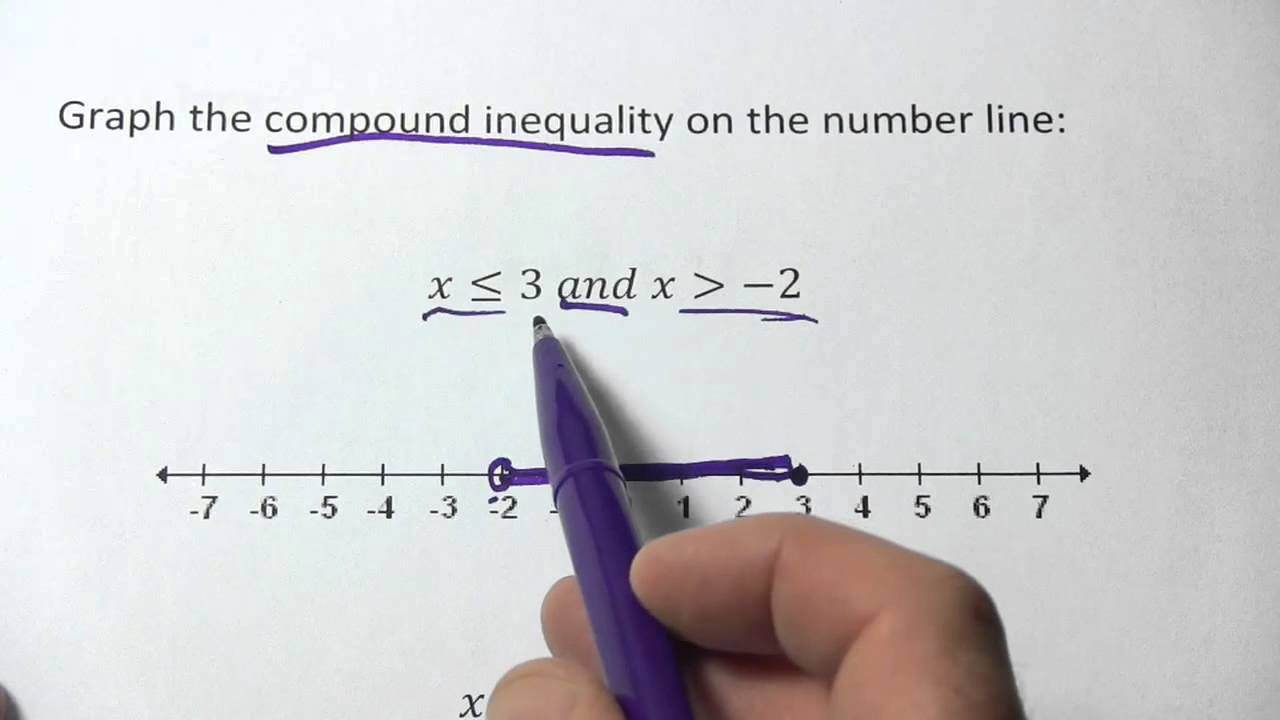# Algebra Tutoring for Graphing Compound Inequalities

Once algebra and pre-algebra students in Los Alamitos have mastered solving and then graphing simple inequalities on a number line, the next step is solving compound inequalities and being able to graph these more complicated equations.Once algebra and pre-algebra students in Los Alamitos have mastered solving and then graphing simple inequalities on a number line, the next step is solving compound inequalities and being able to graph these more complicated equations.

## Understanding Compound Inequalities

An outstanding algebra tutor can explain that compound inequalities are two inequalities, graphed on the same number line, that are linked with “and” or “or.”

In algebra, a compound inequality is a conjunction of two inequalities.  An example of algebraic expressions showing compound inequalities is:

• 3  <  x  <  7 (this is an “and” form of compound inequality.  The value for X has to be less than seven AND greater than three. Any value between three and seven will satisfy the equation.)

Another example for graphing compound inequalities in algebra is:

• x <  -2 or x  >  6 (this is an “or” form of compound inequality.  The value for X can be less than negative two OR greater than 6. X cannot be any value between negative two and six.)

Compound inequalities can come in multiple forms. This might be difficult for algebra students to understand, but a good algebra tutor can provide multiple examples to aid the students to grasp these expressions better.

These algebraic expressions can occur when there are absolute value problems involving inequalities.  An algebra tutor will also let you know that they occur when two conditions need to be accounted for in a word problem, such as a minimum weight and maximum weight, a minimum speed and a maximum speed, or a lowest and highest elevation.  In each of these conditions, a good algebra student should look for the words AND or OR to see which type of compound inequality it is.

Now, look at the image at the top of the post.  The difference between AND and OR inequalities is quite clear.  AND inequalities go toward each other; that is, they form a line segment between two points.  OR inequalities, on the other hand, go away from each other.  They form two rays, with a space in between that cannot be satisfied by either algebraic equation in the inequality.

Getting Help in Algebra

If your student is having a hard time understanding algebra, Firefly Tutors offers algebra tutoring to increase understanding and improve grades.

We have excellent algebra tutors that will come to your home in Los Alamitos, Anaheim, Seal Beach, and throughout Orange County.

We have tutors in every subject, both in Los Alamitos and beyond.